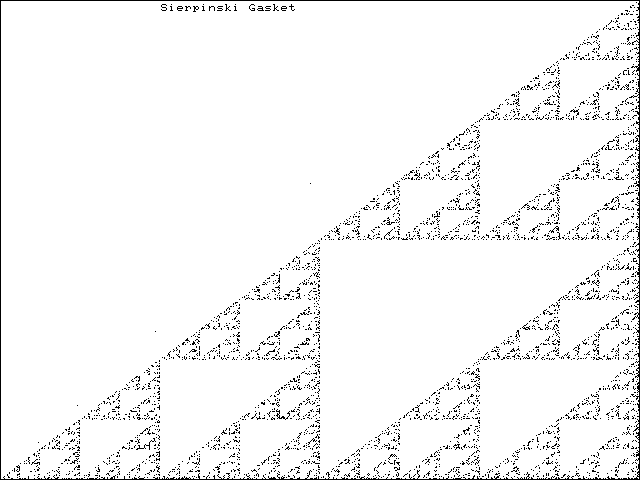Return

```                    PASCAL'S TRIANGLE

Z<-PASCAL N;T
T<-1 & Z<-""
AA: ->(0=N--)/0 & Z<-Z,:(-36-4*rT)m72t8zT & T<-(0,T)+T,0 & ->AA

\$ PASCAL 9
1
1       1
1       2       1
1       3       3       1
1       4       6       4       1
1       5      10      10       5       1
1       6      15      20      15       6       1
1       7      21      35      35      21       7       1
1       8      28      56      70      56      28       8       1

When the values are coloured according to whether they are even or
odd you get a series of increasing triangles called the Sierpinski

This may be generated by a random walk in the complex plane:-

Z[n+1] <-  (Z[n]+X[n])/2

where X,X,... are random variables, each taking one of
the three values a,b,c with probability 1/3. Here (a,b,c) are the
vertices of a triangle.
```
The generation of this triangle is very easy to rotate. It is also possible to generalise the procedure to four or more points. The formula should be:-

```                Z[n+1]<-(Z[n]+(n-2)*X[n])/(n-1)
```
Here X is a random variable which takes one of n complex values with probability 1/n.

When done on a computer the random walks are completely determined. each X[n] is determined by its precedessor. The pair (Z,X) satisfies an equation (Z,X)<-F(Z,X). This is an example of a dynamical system. The random variable X is often generated by modular arithmetic.

## SIERPINSKI TRIANGLE#### SOURCE CODE

The dynamical systems for triangles and Cantor Dust are very similar. The example program draws a rectangle, designates a random triangle then tiles the interior with random dots selected by going half way to a randomly selected vertex. Integer co-ordinates are used throughout, and the computation is simple but laborious since each step depends on the previous value calculated.

Z<-TRIANGLES STR;NLINES;DZ;SI;T;ZT |Display triangles AA: 7 #kjplot \$GW & 0 #kjbloc \$GW+1 1 _2 _2 DZ<-(1 #theta Z)-Z<-(3 2 r \$GW[0 1])+? 3 2 r \$GW[2 3] SI<-1+? 15 & \$GZ<-Z 0 r SI #kjvec DZ SI SP_TILE Z WAIT \$DELAY ->AA XX:Z<-"" Z<-SI SP_TILE A;CNT;T;N;N2;N1 |Tile a figure with sierpinski gasket or cantor dust T<-i0 & N<-1tr:A & Z<- (+/A)%N & N1<-N-1 & N2<-N-2 & CNT<-12000 AA: ->(0=CNT--)/XX T<-T,:Z<-(Z+N2*A[?N])%N1 & ->(500>1tr:T)/AA SI #kjplot T+(rT)r\$GW[0 1] & T<-i0 ->AA XX:Z<-""
Back to the Top